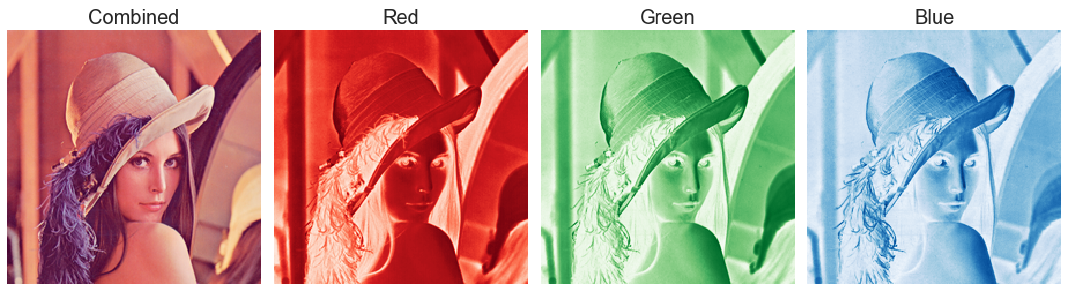# 在Python中使用K-Means聚类和PCA主成分分析进行图像压缩

# image processing
from PIL import Image
from io import BytesIO
import webcolors

# data analysis
import math
import numpy as np
import pandas as pd

# visualization
import matplotlib.pyplot as plt
from mpl_toolkits import mplot3d
import seaborn as sns

# modeling
from sklearn.cluster import KMeans
from sklearn.decomposition import PCA
from sklearn.preprocessing import MinMaxScaler

## 探索图像ori_img = Image.open("images/lena.png")
ori_imgX = np.array(ori_img.getdata())
ori_pixels = X.reshape(*ori_img.size, -1)
ori_pixels.shape

def imageByteSize(img):
img_file = BytesIO()
image = Image.fromarray(np.uint8(img))
image.save(img_file, 'png')
return img_file.tell()/1024
ori_img_size = imageByteSize(ori_img)
ori_img_n_colors = len(set(ori_img.getdata()))


lena.png的原始图像大小为86 KB，并具有37270种独特的颜色。 因此，我们可以说lena.png中的两个像素具有相同的精确RGB值的可能性很小。ori_img_total_variance = sum(np.linalg.norm(X - np.mean(X, axis = 0), axis = 1)**2)posted @ 2020-04-09 13:43  deephub  阅读(554)  评论(0编辑  收藏